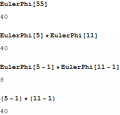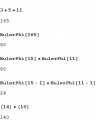# Relatively Prime

#### zulfi100

Joined Jun 7, 2012
656
Hi,
One method to find relative prime numbers for a given N is:
RP(55) = RP(5-1) * RP(11-1)
= 4 * 10
40.

Is the above a correct method, somebody please guide me.

Zulfi.

#### WBahn

Joined Mar 31, 2012
26,326
Huh???

Clearly 40 and 55 are NOT relatively prime (they are both divisible by 5).

#### zulfi100

Joined Jun 7, 2012
656
Hi,
Does it say that there are 40 numbers relatively prime to 55? What does it tell us?

Zulfi.

#### WBahn

Joined Mar 31, 2012
26,326
Ah, it sounded like you were saying that you were using that method to find a number that was relatively prime to 55, but I see that you meant that you were finding how many numbers are relatively prime to 55 -- also known as Euler's totient function. Yes, what you showed is correct in the special case of a number that is a product of prime numbers, none of which are repeated.

#### The Electrician

Joined Oct 9, 2007
2,803
Hi,
One method to find relative prime numbers for a given N is:
RP(55) = RP(5-1) * RP(11-1)
= 4 * 10
40.

Is the above a correct method, somebody please guide me.

Zulfi.
Assuming that the function RP( ) is the Totient function, the method shown doesn't seem to work:RP(55) = RP(5-1) * RP(11-1) needs to be RP(55) = (5-1) * (11-1)

------------------------------------------------------
Furthermore, not all numbers of the class described by WBahn: a "number that is a product of prime numbers, none of which are repeated. "
work with the method the TS describes:#### WBahn

Joined Mar 31, 2012
26,326
I didn't look closely enough. I was too focused on the steps and not what was actually written.

You are correct, it should be

RP(p1·p2·...·pn) = RP(p1)·RP(p2)·...·RP(pn)

if all of the p's are prime.

#### WBahn

Joined Mar 31, 2012
26,326
Assuming that the function RP( ) is the Totient function, the method shown doesn't seem to work:

View attachment 193613

RP(55) = RP(5-1) * RP(11-1) needs to be RP(55) = (5-1) * (11-1)

------------------------------------------------------
Furthermore, not all numbers of the class described by WBahn: a "number that is a product of prime numbers, none of which are repeated. "
work with the method the TS describes:

View attachment 193614
I'm not following how this is a counter example since 15 is not prime, and so you have

EulerPhi = Euler*EulerPhi = EulerPhi*EulerPhi*EulerPhi = (3-1)(5-1)(11-1) = 2·4·10 = 80

#### The Electrician

Joined Oct 9, 2007
2,803
I'm not following how this is a counter example since 15 is not prime, and so you have

EulerPhi = Euler*EulerPhi = EulerPhi*EulerPhi*EulerPhi = (3-1)(5-1)(11-1) = 2·4·10 = 80
The TS showed a number (55) factored into two factors. He didn't say that the factors had to be individual prime factors of the starting number. I factored my example number (165) into two factors, one of which could be factored further, but nothing in post #1 said that the method being illustrated required it. It appeared that a procedure applied to the two factors would give the desired result. The method was not completely specified.

#### zulfi100

Joined Jun 7, 2012
656
Hi,
phi(37) = 37-1 = 36
phi(21) is not a prime= phi (7) * phi(3) : Not both 7 & 3 are prime = 6 * 2 = 12

I think this is the example which I saw in the book. So 40 is correct.

Zulfi.

#### The Electrician

Joined Oct 9, 2007
2,803
phi(21) is not a prime= phi (7) * phi(3) : Not both 7 & 3 are prime = 6 * 2 = 12
Both 7 & 3 are prime.

Did you mean to say: Now both 7 & 3 are prime = 6 * 2 = 12